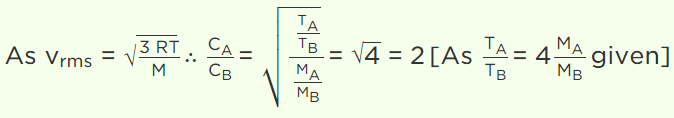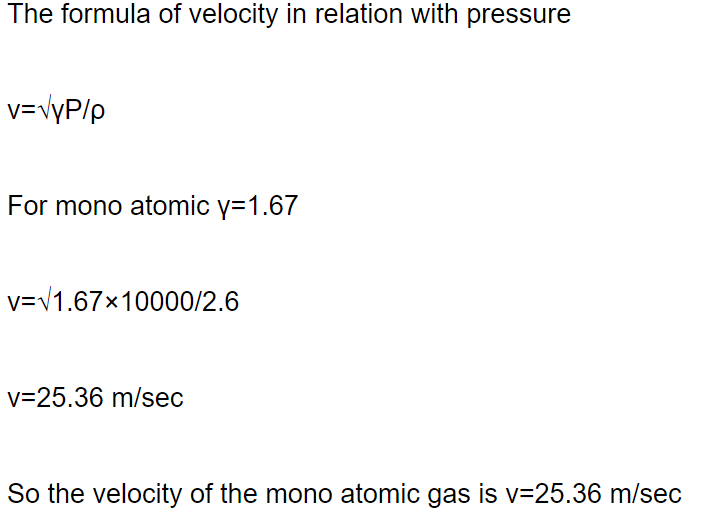## Kinetic Theory Questions and Answers Part-15

1. Let A and B the two gases and given : $\frac{T_{A}}{M_{A}}=4\frac{T_{B}}{M_{B}}$   where T is the temperature and M is molecular mass. If $C_{A}$  and $C_{B}$  are the r.m.s. speed, then the ratio $\frac{C_{A}}{C_{B}}$ will be equal to
a) 2
b) 4
c) 1
d) 0.5

Explanation:2. For a gas at a temperature T the root-meansquare velocity , $V_{rms}$ the most probable speed , $V_{mp}$ and the average speed $V_{av}$ obey the relationship
a) $V_{av} >V_{rms}>V_{mp}$
b) $V_{rms} >V_{av}>V_{mp}$
c) $V_{mp} >V_{av}>V_{rms}$
d) $V_{mp} >V_{rms}>V_{av}$

Explanation: $V_{rms} >V_{av}>V_{mp}$

3. If $V_{H}, V_{N}$  and $V_{O}$  denote the root-mean square velocities of molecules of hydrogen, nitrogen and oxygen respectively at a given temperature, then
a) $V_{N} >V_{O}> V_{H}$
b) $V_{H} >V_{N}> V_{O}$
c) $V_{O} =V_{N}= V_{H}$
d) $V_{O} >V_{H}> V_{N}$

Explanation: $V_{H} >V_{N}> V_{O}$

4. If mass of He atom is 4 times that of hydrogen atom then mean velocity of He is
a) 2 times of H-mean value
b) 1/2 times of H-mean value
c) 4 times of H-mean value
d) Same as H-mean value

Explanation: If mass of He atom is 4 times that of hydrogen atom then mean velocity of He is 1/2 times of H-mean value

5. The r.m.s. speed of a group of 7 gas molecules having speeds (6, 4, 2, 0, – 2, – 4, – 6) m/s is
a) 1.5 m/s
b) 3.4 m/s
c) 9 m/s
d) 4 m/s

Explanation:6. If the ratio of vapour density for hydrogen and oxygen is $\frac{1}{16}$ , then under constant pressure the ratio of their rms velocities will be
a) $\frac{4}{1}$
b) $\frac{1}{4}$
c) $\frac{1}{16}$
d) $\frac{16}{1}$

Explanation:7. Molecular motion shows itself as
a) Temperature
b) Internal Energy
c) Friction
d) Viscosity

Explanation: Molecular motion shows itself as temperature

8. According to the kinetic theory of gases, at absolute temperature
a) Water freezes
b) Liquid helium freezes
c) Molecular motion stops
d) Liquid hydrogen freezes

Explanation: Molecular motion stops

9. The r.m.s. speed of gas molecules is given by
a) $2.5\sqrt{\frac{RT}{M}}$
b) $1.73\sqrt{\frac{RT}{M}}$
c) $2.5\sqrt{\frac{M}{RT}}$
d) $1.73\sqrt{\frac{M}{RT}}$

Explanation: The r.m.s. speed of gas molecules is given by $1.73\sqrt{\frac{RT}{M}}$
10. What is the velocity of wave in monoatomic gas having pressure 1 kilopascal and density $2.6kg\diagup m^{3}$
b) $8.9\times10^{3}m\diagup s$